# Module 7: Graphs, Part I

#### What is a Graph?

In-Class Exercise 7.0: Let's start with a simple exercise: The Calling Circle Problem.

Informal definition:

• A graph is a mathematical abstraction used to represent "connectivity information".

• A graph consists of vertices and edges that connect them, e.g.,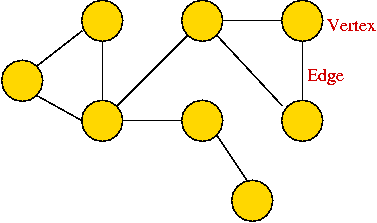• It shouldn't be confused with the "bar-chart" or "curve" type of graph.

Formally:

• A graph G = (V, E) is:
• a set of vertices V
• and a set of edges E = { (u, v): u and v are vertices }.

• Two types of graphs:
• Undirected graphs: the edges have no direction.
• Directed graphs: the edges have direction.

• Example: undirected graph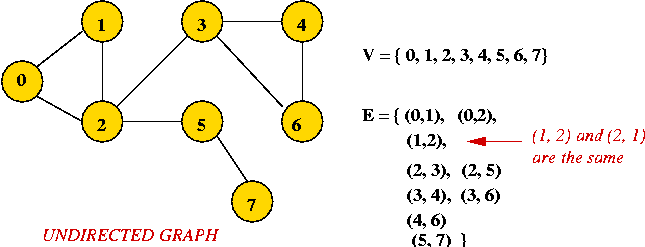• Edges have no direction.
• If an edge connects vertices 1 and 2, either convention can be used:
• No duplication: only one of (1, 2) or (2, 1) is allowed in E.
• Full duplication: both (1, 2) and (2, 1) should be in E.

• Example: directed graph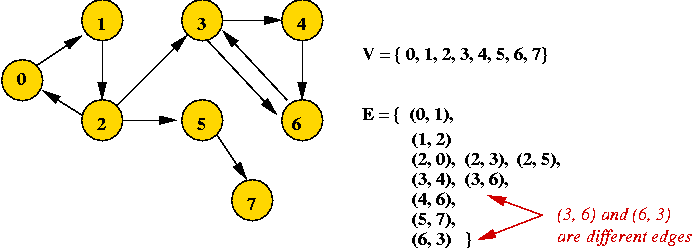• Edges have direction (shown by arrows).
• The edge (3, 6) is not the same as the edge (6, 3) (both exist above).

Depicting a graph:

• The picture with circles (vertices) and lines (edges) is only a depiction
=> a graph is purely a mathematical abstraction.

• Vertex labels:
• Can use letters, numbers or anything else.
• Convention: use integers starting from 0.
=> useful in programming, e.g. degree[i] = degree of vertex i.

• Edges can be drawn "straight" or "curved".

• The geometry of drawing has no particular meaning: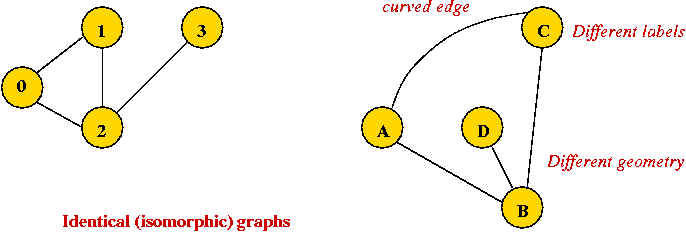Graph conventions:

• What's allowed (but unusual) in graphs:
• Self-loops (occasionally used).
• Multiple edges between a pair of vertices (rare).
• Disconnected pieces (frequent in some applications).
Example: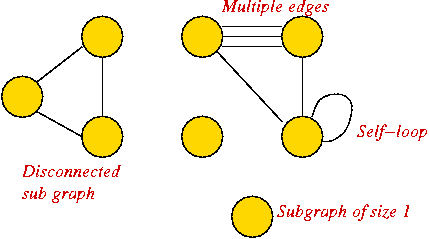• What's not (conventionally) allowed:
• Mixing undirected and directed edges.
• Re-using labels in vertices.
• Bidirectional arrows.

• Most common:
• No multiple edges.
• No self-loops.

• Other terms used:
• Vertices: nodes, terminals, endpoints.
• Edges: links, arcs.
In-Class Exercise 7.1: If we disallow multiple edges and self-loops, what is the maximum number of edges in an undirected graph with n vertices? What is this number in order-notation?

Definitions:

• Degrees:
• Undirected graph: the degree of a vertex is the number of edges incident to it.
• Directed graph: the out-degree is the number of (directed) edges leading out, and the in-degree is the number of (directed) edges terminating at the vertex.

• Neighbors:
• Two vertices are neighbors (or are adjacent) if there's an edge between them.
• Two edges are neighbors (or are adjacent) if they share a vertex as an endpoint.

• Paths:
• Undirected: a sequence of vertices in which successive vertices are adjacent.
• Directed: a sequence of vertices in which every pair of successive vertices has this property: there's a directed edge from the first to the second.
• A simple path does not repeat any vertices (and therefore edges) in the sequence.
• A cycle is a simple path with the same vertex as the first and last vertex in the sequence.

• Connectivity:
• Undirected: Two vertices are connected if there is a path that includes them.
• Directed: Two vertices are strongly-connected if there is a (directed) path from one to the other.

• Components:
• A subgraph is a subset of vertices together with the edges from the original graph that connects vertices in the subset.
• Undirected: A connected component is a subgraph in which every pair of vertices is connected.
• Directed: A strongly-connected component is a subgraph in which every pair of vertices is strongly-connected.
• A maximal component is a connected component that is not a proper subset of another connected component.

• Digraph: another name for a directed graph.

Example: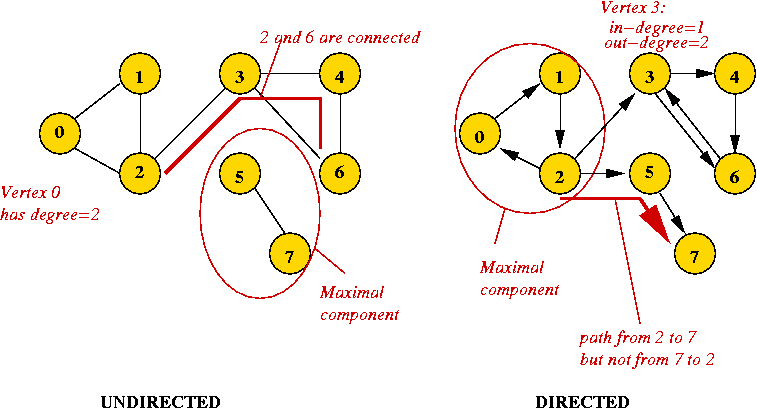More definitions:

• Euler tour: A cycle that traverses all edges exactly once (but may repeat vertices).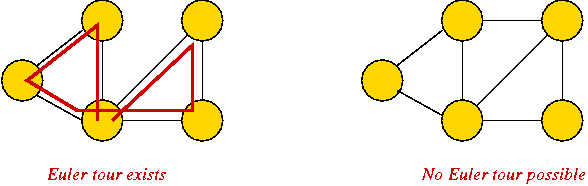Known result: Euler tour exists if and only if all vertices have even degree.

• Hamiltonian tour: A cycle that traverses all vertices exactly once.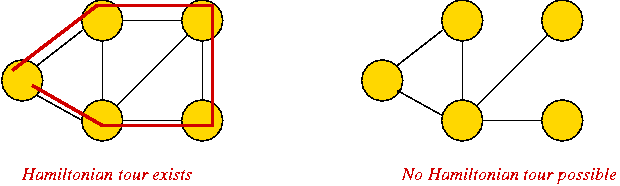Known result: testing existence of a Hamiltonian tour is (very) difficult.

• Euler path: A path that traverses all edges exactly once.

• Hamiltonian path: A path that traverses all vertices exactly once.

• Trees:
• A tree is a connected graph with no cycles.
• A spanning tree of a graph is a connected subgraph that is a tree.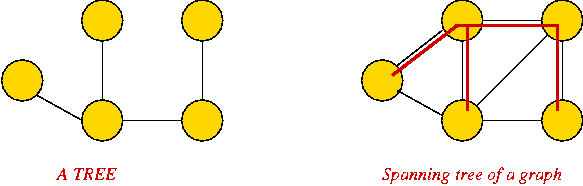• Weighted graphs:
• Sometimes, we include a "weight" (number) with each edge.
• Weight can signify length (for a geometric application) or "importance".
• Example: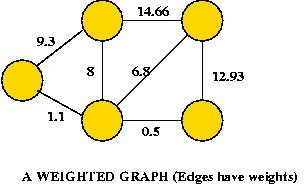Why are graphs important?

• History:
• Maze-searching.
• Euler's crossing problem: the Konigsberg bridges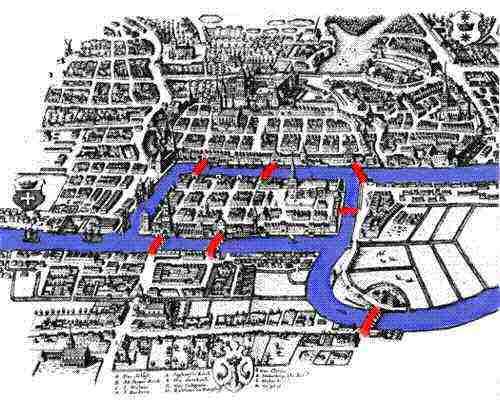How to cross each bridge just once and return to starting point?

• Applications:
• Fundamental mathematical construct to represent "connectivity".
• Appears in thousands of problems.
• Source of many classic problems: traveling salesman, routing, spanning trees.
• Many "graph-structured" applications: networks, transportation-systems, electronic circuits, molecules.

• Source of theory:
• Many important algorithms.
• Key to understanding algorithm design and analysis.
• Simple to describe, yet perplexing:
• Euler tour: easy problem.
• Hamiltonian tour: hard problem.

The field of graph theory:

• Large area of mathematics:
• Analysis of general graphs.
• Analysis of special types of graphs.
• Many classic problems
e.g., the four-color theorem.
• Optimization problems based on graphs,
e.g., shortest-paths.

• Graph algorithms: an area in computer science.
• Rich source of algorithms, theory, insight.
• Useful algorithms used in many applications
(e.g., in a compiler).

In this course:

• Fundamental algorithms for exploring a graph: breadth-first and depth-first.
• Finding shortest paths and minimum spanning tree.
• Convention about undirected vs. directed:
• When not specified, assume undirected.
• Unless otherwise mentioned, an algorithm or definition about undirected graphs usually can be modified to apply to directed graphs.
(although, directed graphs are usually more complicated).
In-Class Exercise 7.2: Suppose di is the degree of vertex i in a connected undirected graph with n vertices and m edges. Let D = d1 + ... + dn. What is the relation between D and m?

#### Graph Data Structures

First, an idea that doesn't work:

• We have already represented trees (like binary trees) with node instances and pointers between instances.

• Idea: use a node instance for each vertex, and a pointer from one vertex to another if an edge exists between them.

In-Class Exercise 7.3: Why doesn't it work? The two fundamental data structures:

• Key idea: use a 2D matrix.
• Row i has "neighbor" information about vertex i.
• Undirected: adjMatrix[i][j] = 1 if and only if there's an edge between vertices i and j.
adjMatrix[i][j] = 0 otherwise.
• Directed: adjMatrix[i][j] = 1 if and only if there's an edge from i to j.
adjMatrix[i][j] = 0 otherwise.
• Example: undirected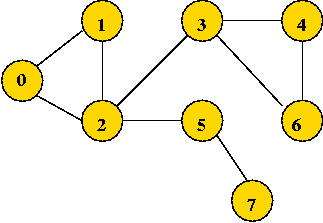```
0 1 1 0 0 0 0 0
1 0 1 0 0 0 0 0
0 1 0 1 0 1 0 0
0 0 1 0 1 0 1 0
0 0 0 1 0 0 1 0
0 0 1 0 0 0 1 1
0 0 0 1 1 0 0 0
0 0 0 0 0 1 0 0
```

Note: adjMatrix[i][j] == adjMatrix[j][i] (convention for undirected graphs).

• Example: directed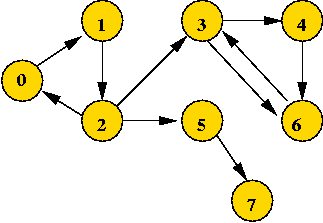```
0 1 0 0 0 0 0 0
0 0 1 0 0 0 0 0
1 0 0 1 0 1 0 0
0 0 0 0 1 0 1 0
0 0 0 0 0 0 1 0
0 0 1 0 0 0 0 1
0 0 0 1 0 0 0 0
0 0 0 0 0 0 0 0
```

• Key idea: use an array of vertex-lists.
• Each vertex list is a list of neighbors.
• Example: undirected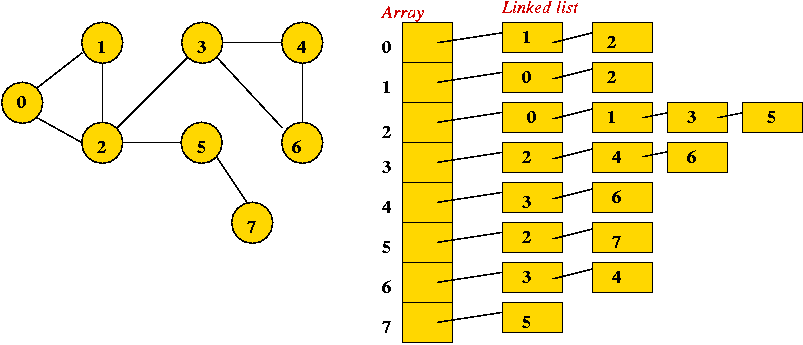• Example: directed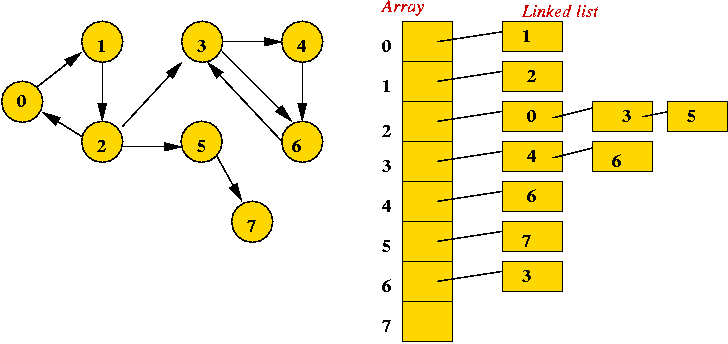• Convention: in each list, keep vertices in order of insertion
=> add to rear of list

• Both representations allow complete construction of the graph.

• Advantages of matrix:
• Simple to program.
• Some matrix operations (multiplication) are useful in some applications (connectivity).
• Efficient for dense (lots of edges) graphs.

• Less storage for sparse (few edges) graphs.
• Easy to store additional information in the data structure.
(e.g., vertex degree, edge weight)

In-Class Exercise 7.4: Suppose a graph has V vertices and E edges. In order-notation (in terms of V and E), what is the size of the adjacency matrix representation? What is the size of the adjacency list representation?

In-Class Exercise 7.5: Write a Java class to implement the adjacency list representation. Do not write all the details, but merely what you need for initializing the data structure.

• ADT = Abstract Data Type.

• What operations should a graph ADT support?
• Insertion of vertices, edges.
• Is the graph connected?
• What are the connected components?
• Does the graph have a cycle?

• Example: consider this interface for an undirected graph ADT:
```
public interface UndirectedGraphSearchAlgorithm {

// Call this once to set up data structures.

public void initialize (int numVertices, boolean isWeighted);

// Call this repeatedly with each edge to be inserted:

public void insertUndirectedEdge (int startVertex, int endVertex, double weight);

// Return the number of connected components. If "1", then the whole
// graph is connected.

public int numConnectedComponents ();

// Return the component to which each vertex belongs.

public int[] componentLabels ();

// Is there a cycle in the graph?

public boolean existsCycle();

}
```

In-Class Exercise 7.6: How would you use this ADT to tell whether a graph is a connected tree?

Next, let's look at some sample code using the matrix representation:

• Convention for weighted graphs: use weight=0 to represent the lack of an edge.
```
public class UndirectedGraphSearch implements UndirectedGraphSearchAlgorithm {

int numVertices;                 // Number of vertices, given as input.
int numEdges;                    // We keep track of the number of edges.

boolean isWeighted;              // Is this a weighted graph?

double [][] adjMatrix;           // The matrix. Note: we use "double" to store
// "double" weights, if the graph is weighted.

public void initialize (int numVertices, boolean isWeighted)
{
// Store:
this.numVertices = numVertices;
this.isWeighted = isWeighted;

if (useMatrix) {

// Create the adjacency matrix.
adjMatrix = new double [numVertices][];
for (int i=0; i < numVertices; i++) {
adjMatrix[i] = new double [numVertices];
for (int j=0; j < numVertices; j++)
}
}
else {

// Adjacency-list representation ...

}

numEdges = 0;
}

// Insert a given input edge.

public void insertUndirectedEdge (int startVertex, int endVertex, double weight)
{
if (useMatrix) {

// Unweighted graph: use weight 1.0.
if (! isWeighted) {

// Insert in both places:
adjMatrix[endVertex][startVertex] = 1.0;   // Remove this for directed graphs

}
else {

// Use weight ...

}

numEdges ++;
return;

} // end-use-Matrix.
else {

}

}
```

The same with an adjacency list:

```
public class UndirectedGraphSearch implements UndirectedGraphSearchAlgorithm {

int numVertices;                 // Number of vertices, given as input.
int numEdges;                    // We keep track of the number of edges.

boolean isWeighted;              // Is this a weighted graph?

boolean useMatrix = false;       // Adjacency-matrix or list?

LinkedList[] adjList;            // The list (of lists): one list for each vertex.
// We are using java.util.LinkedList as our linked list.
// This will store instances of GraphEdge.

// We will test new insertions to see if they exist. Because LinkedList performs
// ".equals()" testing for containment, we need an instance (and only one) of GraphEdge
// for such testing.
GraphEdge testEdge = new GraphEdge (-1, -1, 0);

public void initialize (int numVertices, boolean isWeighted)
{

// Store:
this.numVertices = numVertices;
this.isWeighted = isWeighted;

if (useMatrix) {

// Adjacency matrix creation ...

}
else {

for (int i=0; i < numVertices; i++)
// Note: we are using java.util.LinkedList.

}

numEdges = 0;

}

// Insert a given input edge.

public void insertUndirectedEdge (int startVertex, int endVertex, double weight)
{
if (useMatrix) {

// ... Matrix representation ...

}
else {

// Adj-list representation: see if the edge is already there.
testEdge.startVertex = startVertex;
testEdge.endVertex = endVertex;

// Exploit the methods in java.util.LinkedList.
if (adjList[startVertex].contains (testEdge)) {
// ... signal error ...
return;
}

// It's undirected, so add to both vertex lists.
GraphEdge e = new GraphEdge (startVertex, endVertex, 1.0);

// We wouldn't have this for directed graphs:
GraphEdge e2 = new GraphEdge (endVertex, startVertex, 1.0);

numEdges ++;

}

}
```

• "Searching" here means "exploring" a particular graph.

• Searching will help reveal properties of the graph
e.g., is the graph connected?

• Usually, the input is: vertex set and edges (in no particular order).

Key ideas in breadth-first search: (undirected)

• Mark all vertices as "unvisited".
• Initialize a queue (to empty).
• Find an unvisited vertex and apply breadth-first search to it.
• In breadth-first search, add the vertex's neighbors to the queue.
• Repeat: extract a vertex from the queue, and add its "unvisited" neighbors to the queue.

Example:

• Initially, place vertex 0 in the queue.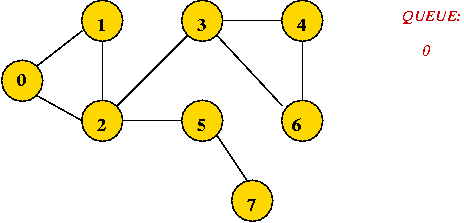• Dequeue 0
=> mark it as visited, and add its unvisited neighbors to queue: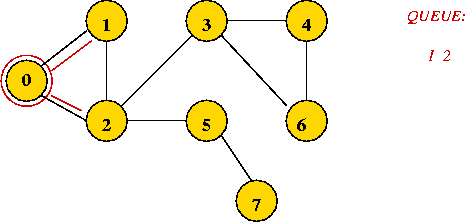• Dequeue 1
=> mark it as visited, and add its unvisited neighbors to queue: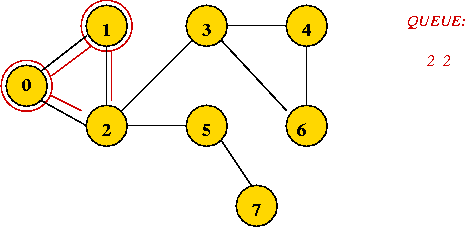• Dequeue 2
=> mark it as visited, and add its unvisited neighbors to queue: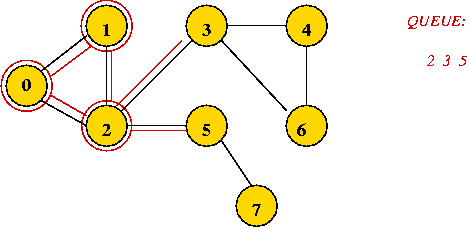• Dequeue 2
=> it's already visited, so ignore.

• Continuing ...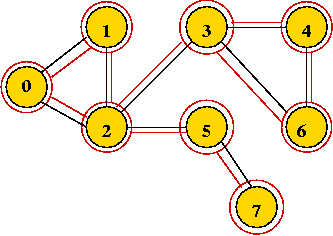• Breadth-first search tree, and visit order: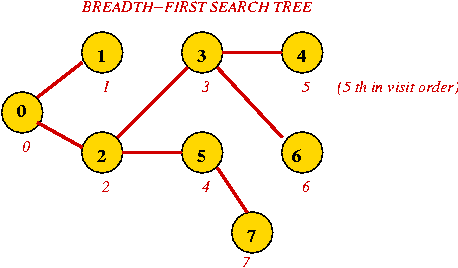• Exploring an edge: examining an unvisited neighbor.
• If an unvisited neighbor gets on the queue for the first time, the edge is called a "tree edge".
• Putting the tree edges and all vertices together results in: the breadth-first search tree.
• For a particular graph and its implementation, the tree produced is unique.
• However, starting from another vertex will result in another tree, that may be just as useful.

In-Class Exercise 7.7: What is the visit-order and breadth-first search tree for this graph: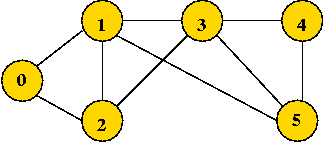Searching an unconnected graph:

• The connected components are explored in order:
• Example: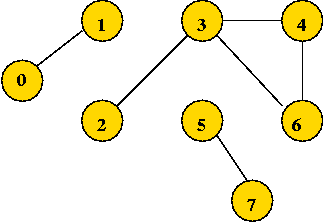The tree, and visit order: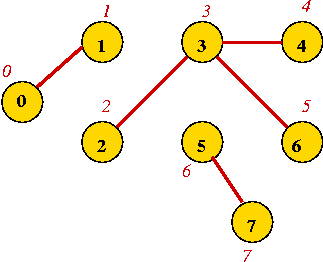Implementation:

• There are two varieties:
• Allow a vertex to have two states: "unvisited" and "visited"
• An "unvisited" vertex can get placed in the queue multiple times.
It's possible to dequeue a "visited" vertex.
• When dequeueing, check whether vertex was visited.
• Allow three states: "unvisited", "in-queue", and "visited":
• When placing a neighbor in the queue for the first time, mark it as "in-queue".
• Place only "unvisited" neighbors in queue.
=> Queue has only unique, unvisited vertices.
• Extra space required.

• We'll use the former approach, with an adjacency matrix:

• Pseudocode:
```
Input: A graph's adjacency matrix, number of vertices n.

// Visit order will start with "0", so initialize to -1.
1.   for i=0 to n-1
2.       visitOrder[i] = -1
3.   endfor

// A counter for the order:
4.   visitCount = -1

// Standard queue data structure.
5.   Create queue;

// Look for an unvisited vertex and explore its tree.
// We need this because the graph may have multiple components.

6.   for i=0 to n-1
7.       if visitOrder[i] < 0
// We call this "iterative" because other searches are recursive.
9.       endif
10.  endfor
```
```
Input: vertex u, adjMatrix is assumed to be global.

// Queue needs to be reset for each tree.
1.   Clear queue;

// Place root of tree on the queue.

// Continue processing vertices until no more can be added.
3.   while queue not empty

// Remove a vertex.
4.       v = remove item at front of queue;
// If it hasn't been visited ...
5.       if visitOrder[v] < 0
// Visit the vertex.
6.         visitCount = visitCount + 1
7.         visitOrder[v] = visitCount
// Look for neighbors to visit.
8.         for i=0 to n-1
9.           if adjMatrix[v][i] = 1 and i != v  // Check self-loop: i != v
11.          endif
12.        endfor
13.      endif

14.  endwhile
```

• A sample Java implementation: (source file)
```
import java.util.*;

public class UndirectedBreadthFirstMatrix {

int numVertices;                 // Number of vertices, given as input.
int numEdges;                    // We keep track of the number of edges.
boolean isWeighted;              // Is this a weighted graph?
boolean useMatrix = true;        // Adjacency-matrix or list?
double [][] adjMatrix;           // The matrix. Note: we use "double" to store
// "double" weights, if the graph is weighted.

int[] visitOrder;                // visitOrder[i] = the i-th vertex to be visited in order.
int visitCount;                  // We will track visits with this counter.
LinkedList queue;                // The queue for breadth-first, a java.util.LinkedList instance.

public void initialize (int numVertices, boolean isWeighted)
{
// ... We've seen this before ...
}

public void insertUndirectedEdge (int startVertex, int endVertex, double weight)
{
// ...
}

//-------------------------------------------------------------------------------

// Initialize visit information before search.

void initSearch ()
{
// IMPORTANT: all initializations will use "-1". We will test
// equality with "-1", so it's important not to change this cavalierly.
visitCount = -1;
for (int i=0; i < numVertices; i++) {
visitOrder[i] = -1;
}
}

// Matrix implementation of breadth-first search.

{
// 1. Initialize visit variables.
initSearch ();

// 2. Create a queue.
queue = new LinkedList();

// 3. Find an unvisited vertex and apply breadth-first search to it.
//    Note: if the graph is connected, the call with i=0 will result
//          in visiting all vertices. Nonetheless, we don't know this
//          in advance, so we need to march through all the vertices.

for (int i=0; i < numVertices; i++) {
if (visitOrder[i] == -1) {
// We call it "iterative" because depthFirst search is "recursive".
}
}

}

// Apply breadthfirst search to a particular vertex (root of a tree)

void breadthFirstMatrixIterative (int u)
{
// 1. A fresh queue is used for a new breadth-first search.
queue.clear();

//    Because LinkedList stores objects, we "objectify" the vertex. A more efficient
//    implementation would use a separate queue of int's.

// 3. As long as the queue has vertices to process...
while (! queue.isEmpty()) {

// 3.1 Dequeue and extract vertex using java.util.LinkedList.removeFirst().
Integer V = (Integer) queue.removeFirst();
int v = V.intValue();

// 3.2 If the vertex has been visited, skip.
if (visitOrder[v] != -1)
continue;

// 3.3 Otherwise, set its visit order.
visitCount++;
visitOrder[v] = visitCount;

// 3.4 Next, place its neighbors on the queue.
for (int i=0; i < numVertices; i++) {
// 3.4.1 First, check whether vertex i is a neighbor.
if ( (adjMatrix[v][i] > 0) && (i != v) ) {

// 3.4.1.1 If i hasn't been visited, place in queue.
if (visitOrder[i] == -1) {
}
}

} // end-for

} // end-while

}

} // end-class
```

• Assume V vertices and E edges.

• Each vertex is processed V times (worst-case) in starting a tree.
=> O(V).

• Searching for a neighbor: O(V) (scan through matrix).
=> all scans take O(V2).

• Each queue operation is O(1).

• Each edge is processed once:
=> O(E) queue operations and O(E) vertex manipulations.

• Total: O(V2 + E) = O(V2).

• Searching for a neighbor: O(# neighbors)
=> total neighbor searches is O(E) (Why?)

• Other operations are the same
Total: O(V + E) = O(E).

In-Class Exercise 7.8: Which is better: adjacency matrix or adjacency list?

About O(V + E):

• Note that O(V + E) = O(E)
=> it is written as O(V + E) just for emphasis.

• O(V + E) is optimal:
• Every vertex and every edge must be examined.
=> O(V + E)
=> not possible to do better than O(V + E)

• BFS (with adjacency list) is an example of an optimal algorithm.

Directed graphs:

• BFS in directed graphs is similar, except that a vertex's "neighbor" is one that's reachable by an edge going out from the vertex.
=> search code is identical (but insertion code is different).

Applications:

• Connectivity:
• Breadth-first search identifies connected components.
• However, depth-first search is preferred (required for directed graphs).
• Shortest paths:
• A path between two vertices in the tree is the shortest path in the graph.
• Optimization algorithms:
• Various problems result in "graph search space".
• BFS together with "exploration rules" is often used to search for solutions (e.g., branch-and-bound exploration).

Note: BFS works on a weighted graph by ignoring the weights and only using connectivity information (i.e., is there an edge or not?).

#### Depth-First Search on Undirected Graphs

Key ideas:

• Mark all vertices as "unvisited".
• Visit first vertex.
• Recursively visit its "unvisited" neighbors.

Example:

• Start with vertex 0 and mark it visited.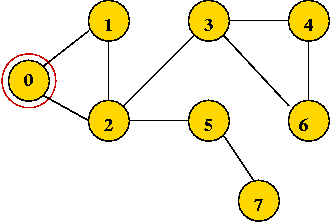• Visit the first neighbor 1, mark it visited.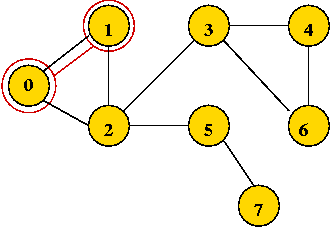• Explore 1's first neighbor, 2.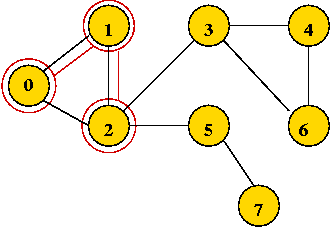• Continuing until all vertices are visited ...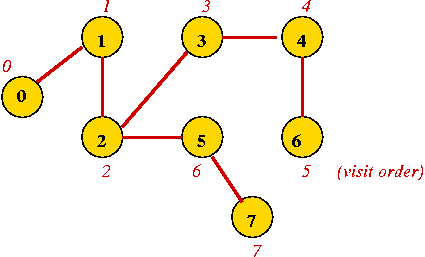• Vertices are marked in order of visit.
• An edge to an unvisited neighbor that gets visited next is in the depth-first search tree.
In-Class Exercise 7.9: What is the visit-order and depth-first search tree for this graph:Implementation:

• Easier than breadth-first search.

• Pseudocode: adjacency matrix
```
Algorithm: depthFirstMatrix (adjMatrix, n)
Input: A graph's adjacency matrix, number of vertices n.

// Visit order will start with "0", so initialize to -1.
1.   for i=0 to n-1
2.       visitOrder[i] = -1
3.   endfor

// A counter for the order:
4.   visitCount = -1

// Look for an unvisited vertex and explore its tree.
// We need this because the graph may have multiple components.
5.   for i=0 to n-1
6.       if visitOrder[i] < 0
7.         depthFirstMatrixRecursive (i)
8.       endif
9.   endfor
```
```
Algorithm: depthFirstMatrixRecursive (v)
Input: vertex v, adjMatrix is assumed to be global.

// Mark vertex v as visited.
1.   visitCount = visitCount + 1
2.   visitOrder[v] = visitCount

// Look for first unvisited neighbor.
3.   for i=0 to n-1
4.       if adjMatrix[v][i] > 0 and i != v
5.           if visitOrder[i] < 0
// If unvisited visit recursively.
6.               depthFirstMatrixRecursive (i)
7.           endif
8.       endif
9.   endfor
```

• Completion order:
• In breadth-first search, once a vertex is processed, it is never processed again.

• In depth-first, we also encounter a vertex after returning from the recursive call.
=> we can record a completion order.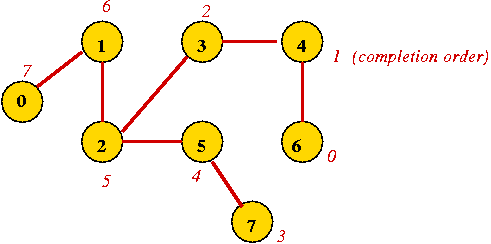• To record the completion order, simply increment the completion-counter after the recursion:
```
Algorithm: depthFirstMatrixRecursive (v)
Input: vertex v, adjMatrix is assumed to be global.

// Mark vertex v as visited.
1.   visitCount = visitCount + 1
2.   visitOrder[v] = visitCount

// Look for first unvisited neighbor.
3.   for i=0 to n-1
4.       if adjMatrix[v][i] > 0 and i != v  // Check self-loop.
5.           if visitOrder[i] < 0
// If unvisited visit recursively.
6.               depthFirstMatrixRecursive (i)
7.           endif
8.       endif
9.   endfor

// After returning from recursion, set completion order:
10.  completionCount = completionCount + 1
11.  completionOrder[v] = completionCount
```

• Note: completionCount and completionOrder need to be initialized (not shown) in depthFirstMatrix.

• Sample Java code: (source file)
```
public class UndirectedDepthFirstMatrix {

int numVertices;                 // Number of vertices, given as input.
int numEdges;                    // We keep track of the number of edges.
boolean isWeighted;              // Is this a weighted graph?
boolean useMatrix = true;        // Adjacency-matrix or list?
double [][] adjMatrix;           // The matrix. Note: we use "double" to store
// "double" weights, if the graph is weighted.

int[] visitOrder;                // visitOrder[i] = the i-th vertex to be visited in order.
int visitCount;                  // We will track visits with this counter.
int[] completionOrder;           // completionOrder[i] = the i-th vertex that completed.
int completionCount;             // For tracking.

public void initialize (int numVertices, boolean isWeighted)
{
// ...
}

public void insertUndirectedEdge (int startVertex, int endVertex, double weight)
{
// ...
}

//-------------------------------------------------------------------------------
// DEPTH-FIRST SEARCH

// Initialize visit information before search.

void initSearch ()
{
// IMPORTANT: all initializations will use "-1". We will test
// equality with "-1", so it's important not to change this cavalierly.
visitCount = -1;
completionCount = -1;
for (int i=0; i < numVertices; i++) {
visitOrder[i] = -1;
completionOrder[i] = -1;
}
}

// Matrix implementation of depth-first search.

void depthFirstMatrix ()
{
// 1. Initialize visit variables (same initialization for breadth-first search).
initSearch ();

// 2. Find an unvisited vertex and apply depth-first search to it.
//    Note: if the graph is connected, the call with i=0 will result
//          in visiting all vertices. Nonetheless, we don't know this
//          in advance, so we need to march through all the vertices.
for (int i=0; i < numVertices; i++) {
if (visitOrder[i] < 0) {
depthFirstMatrixRecursive (i);
}
}
}

// Recursive visiting of vertices starting from a vertex.

void depthFirstMatrixRecursive (int v)
{
// 1. First, visit the given vertex. Note: visitCount is a global.
visitCount++;
visitOrder[v] = visitCount;

// 2. Now find unvisited children and visit them recursively.
for (int i=0; i < numVertices; i++) {

// 2.1  Check whether vertex i is a neighbor and avoid self-loops.
if ( (adjMatrix[v][i] > 0) && (i != v) ) {
if (visitOrder[i] == -1) {
// i is an unvisited neighbor.
depthFirstMatrixRecursive (i);
}
}
}

// 3. After returning from recursion(s), set the post-order or "completion" order number.
completionCount++;
completionOrder[v] = completionCount;
}

} // end-class
```

"Back" and "Down" edges:

• Consider the previous example: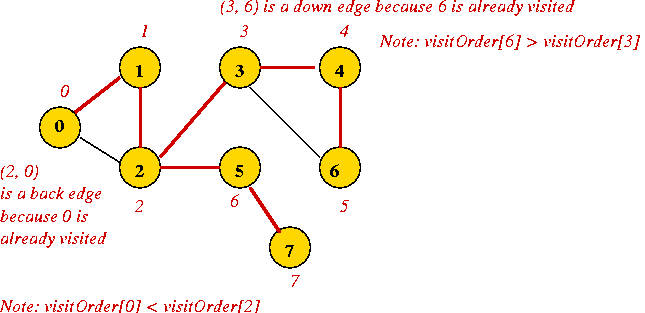• Back edge
• When vertex 2 probes neighbor 0, 0 has already been visited.
• Vertex 0 is higher up in the tree (ancestor of 2)
=> 0 has a lower visitOrder
=> visitOrder < visitOrder.
• The exploration from 2 to 0 is called a back edge.

• Down edge:
• When vertex 3 probes neighbor 6, 6 has already been visited.
• Vertex 6 is lower down in the tree (descendant of 3)
visitOrder > visitOrder
• The exploration from 3 to 6 is called a down edge.

• Let's modify the code to detect back and down edges:
```
Algorithm: depthFirstMatrixRecursive (u, v)
Input: the vertex u from which this is being called, vertex v
to be explored, adjMatrix is assumed to be global.

// Mark vertex v as visited.
1.   visitCount = visitCount + 1
2.   visitOrder[v] = visitCount

// Look for first unvisited neighbor.
3.   for i=0 to n-1
4.       if adjMatrix[v][i] > 0 and i != v  // Check self-loop.

5.           if visitOrder[i] < 0
// If unvisited visit recursively.
6.               depthFirstMatrixRecursive (i)
7.           else if (i != u)  // Avoid trivial case.
8.               if visitOrder[i] < visitOrder[v]
// Found a back edge.
9.                   numBackEdges = numBackEdges + 1
10.              else
// Found a down edge.
11.                  numDownEdges = numDownEdges + 1
12.              endif
13.          endif  // visitOrder < 0

14.       endif  // adjMatrix[v][i] > 0
15.   endfor

// After returning from recursion, set completion order:
16.  completionCount = completionCount + 1
17.  completionOrder[v] = completionCount
```

• Why identify back and down edges?
• A graph with no back edges revealed by DFS is an acyclic graph.
• Down edges are useful in identifying so-called articulation edges.

Identifying connected components:

• Depth-first search is most often used for identifying connected components in an undirected graph.

• Key ideas:
• Every time depth-first is re-started (in depthFirstMatrix)
a new component has been found.
create a new component label.
• In the recursion (depthFirstMatrixRecursive), simply identify each vertex with the current component label.

• Let's re-write (part of) the pseudocode to identify components:
```
Algorithm: depthFirstMatrix (adjMatrix, n)
Input: A graph's adjacency matrix, number of vertices n.

// Visit order will start with "0", so initialize to -1.
1.   for i=0 to n-1
2.       visitOrder[i] = -1
3.   endfor

// A counter for the order:
4.   visitCount = -1
5.   completionCount = -1
6.   currentComponentLabel = -1

// Look for an unvisited vertex and explore its tree.
// We need this because the graph may have multiple components.
7.   for i=0 to n-1
8.       if visitOrder[i] < 0
9.           currentComponentLabel = currentComponentLabel + 1
10.          depthFirstMatrixRecursive (i)
11.      endif
12.  endfor
```
```
Algorithm: depthFirstMatrixRecursive (v)
Input: vertex v, adjMatrix is assumed to be global.

// Mark vertex v as visited.
1.   visitCount = visitCount + 1
2.   visitOrder[v] = visitCount

// Mark the component label
3.   componentLabel[v] = currentComponentLabel

// Look for first unvisited neighbor...
4.   // ... etc (same as earlier version)
```

• Sample Java code: (source file).

• Same as breadth-first search: O(V2)

• Why?
• O(1) work for processing each vertex (except for identifying neighbors).
• O(V) work for identifying neighbors.
=> O(V2) overall.

• Similar analysis (to breadth-first search) gives: O(V + E).
• DFS with adjacency list is optimal.

Applications:

• Connectivity: identifying connected components.
=> which earlier-stated problem would this solve?

• Cycle existence.

• Others: finding articulation edges, vertices, "bipartiteness".

• Identifying equivalence classes

#### Depth-First Search in Directed Graphs

Key ideas:

• A straightforward depth-first search is similar to the undirected version
=> only explore edges going outward from a vertex in a directed graph.
• In addition to "back" and "down" edges, it is useful to identify "cross" edges.

Example:

• Consider: (slightly different from previous example)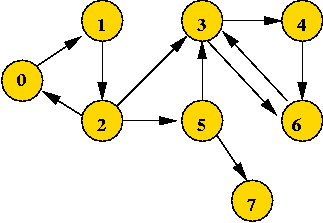• Applying DFS gives: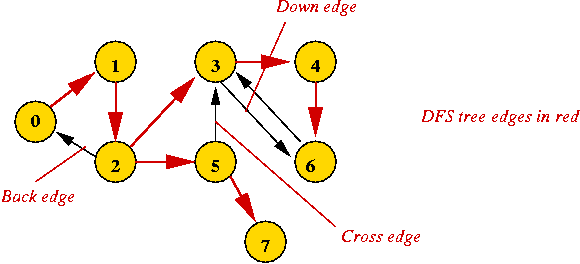Let's modify the pseudocode to detect cross edges as well:

• Key ideas:
• What used to be a back edge in the undirected version is now either a back edge or cross edge.
• Back or down edge: when the vertex on the other side is "visited" but not yet "completed".
• Cross edge: when the vertex on the other side is "completed".

• Pseudocode:
```
Algorithm: depthFirstMatrixRecursive (u, v)
Input: the vertex u from which this is being called, vertex v
to be explored, adjMatrix is assumed to be global.

// Mark vertex v as visited.
1.   visitCount = visitCount + 1
2.   visitOrder[v] = visitCount

// Look for first unvisited neighbor.
3.   for i=0 to n-1
4.       if adjMatrix[v][i] > 0 and i != v  // Check self-loop.

5.           if visitOrder[i] < 0
// If unvisited visit recursively.
6.               depthFirstMatrixRecursive (i)
7.           else if (i != u)  // Avoid trivial case.
8.               if completionOrder[i] < 0    // Not finished processing i yet
// Found a back edge.
9.                   numBackEdges = numBackEdges + 1
10.              else if visitOrder[i] > visitOrder[v]
// Found a down edge.
11.                  numDownEdges = numDownEdges + 1
12.              else
// Found a cross edge
13.                  numCrossEdges = numCrossEdges + 1
14.              endif
15.          endif  // visitOrder < 0

16.       endif  // adjMatrix[v][i] > 0
17.   endfor

// After returning from recursion, set completion order:
18.  completionCount = completionCount + 1
19.  completionOrder[v] = completionCount
```

Analysis: (same as undirected case)

• Adjacency matrix: O(V2).
• Adjacency list: O(V+E).

#### Strongly-Connected Components in Directed Graphs

Finding components:

• Recall, in a directed graph a strongly-connected component is a set of vertices, along with edges associated with those vertices, such that there is a path from every vertex in that set to every other in that set.

• Example: vertices 0,1,2 form a strongly-connected component.In-Class Exercise 7.10: Describe in pseudocode a simple algorithm to find strongly-connected components that uses the above DFS algorithm for directed graphs. Hint: vertices i and j are in the same strongly-connected component if i is reachable from j and j is reachable from i. How long does your algorithm take? We will look at two different algorithms for finding strongly-connected components:
• Kosaraju's Algorithm: this applies DFS twice but in an unusual way.
• Tarjan's Algorithm: computes the components directly but is harder to understand.

Kosaraju's algorithm:

• First, we need to understand the reverse of a directed graph.
• Suppose G = (V,E) is a graph.
• G' = (V,E') is the reverse of G if E'=E with the direction of edges reversed.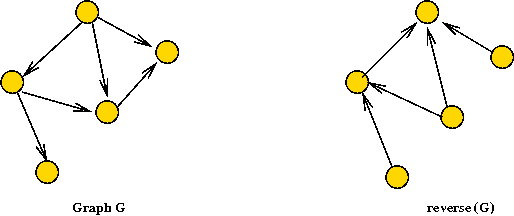• Next, recall completion-order
lower completion order => closer to root

• The algorithm has two phases:
• First phase: find completion order on reverse graph using standard DFS.
• Second phase: use a modified DFS on original graph in which completion order is used as a priority.

• Let's describe the pseudocode first:
```
Algorithm: stronglyConnectedComponents (adjMatrix, n)
Input: A graph's adjacency matrix, number of vertices n.

1.    G' = reverse (G)
2.    completionOrder = depthFirstSearch (G')
3.    sortOrder = sort vertices in reverse order of completion           // Last to first.
4.    componentLabels = modifiedDepthFirstSearch (G, sortOrder)
5.    return componentLabels

Algorithm: modifiedDepthFirstSearch (adjMatrix, sortOrder)
Input: A graph's adjacency matrix, a sort (priority) order of vertices

1.   for i=0 to n-1
2.       visitOrder[i] = -1
3.   endfor
4.   visitCount = -1
5.   currentComponentLabel = -1

// Look for an unvisited vertex and explore its tree.
6.   for i=0 to n-1
7.       v = sortOrder[i]                   // In order of completion of reverse search.
8.       if visitOrder[v] < 0
9.           currentComponentLabel = currentComponentLabel + 1
10.          modifiedDepthFirstMatrixRecursive (v)
11.      endif
12.  endfor
13.  return  componentLabels

Algorithm: modifiedDepthFirstMatrixRecursive (v)

// Mark vertex v as visited and record component label.
1.   visitCount = visitCount + 1
2.   visitOrder[v] = visitCount
3.   componentLabel[v] = currentComponentLabel

// Look for first unvisited neighbor.
4.   for i=0 to n-1
u = sortOrder[i]                   // In order of completion of reverse search.
5.       if adjMatrix[v][u] > 0 and u != v
6.           if visitOrder[u] < 0
7.               depthFirstMatrixRecursive (u)
8.           endif
9.       endif
10.  endfor

```

• Why does this work? The proof is in two parts.

• Consider two vertices u and v.

• Part (1): Suppose u and v are mutually reachable:
• Suppose u is visited before v in the second-phase.
• Then, DFS will certainly visit v.
=> The algorithm reports both in the same component.
• The same holds if v is visited before u.

• Part (2): Suppose u and v are reported in the same component by the algorithm:
• Because u and v are in the same reported component, they are part of a single tree
=> Let w be the root of this tree.
• Then w was visited before u and v.
=> w has a lower completion order than u and v.
(Store this fact for now).
• Next, observe that we reached u from w in the second phase
=> there's a directed path from w to u in G.
=> there's a directed path from u to w in G'.
• Suppose there is no path from w to u in G' (the reverse).
=> Then, the path from u to w in G' would result in u having a higher completion order.
• Thus, there is a path from w to u in G'.
=> There are paths in G from w to u and back.

• The same argument shows that there is path from w to v and back.
=> u and v are mutually reachable in G.

Tarjan's Algorithm:

• Some intuition:
• Consider what happens when we complete processing "3" in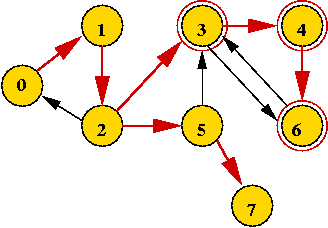• How can we identify the strong component "3, 4, 6"?
• First, there is no back edge that goes from "4, 6" to any node "above" 3.
• Second, both "4" and "6" were visited in the descent from "3".
• But nodes "above" 3 will have a lower visitOrder.
=> keep track of lowest visitOrder reachable by a back edge from a "potential component".
• How to mark vertices in a "component"?
• Build a stack.
• Place vertices on the stack as you recurse down.
• When coming back up the recursion, if you've identified a component, all vertices in the component are going to be at the top of the stack.
• Example above: "3, 4, 6" will be on the stack when returning to "3".
• Another example: "0, 1, 2" will be at the top when returning to "0".
(It won't be removed along the way back from "3" to "0").

• Pseudocode:
```
Algorithm: depthFirstMatrixRecursive (u, v)
Input: the vertex u from which this is being called, vertex v
to be explored, adjMatrix is assumed to be global.

// Mark vertex v as visited.
1.   visitCount = visitCount + 1
2.   visitOrder[v] = visitCount

// Current lowest reachable:
3.   lowestReachable[v] = visitOrder[v];

// Important: minLowestReachable is a local variable so that a
// fresh version is used in each recursive call.
4.   minLowestReachable = lowestReachable[v];

// Initialize stackTop to 0 outside. stackTop points to next available.
5.   stack[stackTop] = v;
6.   stackTop ++;

// Look for first unvisited neighbor.
7.   for i=0 to n-1
8.       if adjMatrix[v][i] > 0 and i != v  // Check self-loop.

9.           if visitOrder[i] < 0
// If unvisited visit recursively.
10.              depthFirstMatrixRecursive (i)
11.          else if (i != u)  // Avoid trivial case.
// Identify back, down and cross edges ... (not shown)
12.          endif  // visitOrder < 0

13.          if lowestReachable[i] < minLowestReachable
14.              minLowestReachable = lowestReachable[i]
15.          endif

16.      endif  // adjMatrix[v][i] > 0
17.   endfor

// We reach here after all neighbors have been explored.
// Check whether a strong component has been found.
18.  if minLowestReachable < lowestReachable[v]
// This is not a component, but we need to update the
// lowestReachable for this vertex since its ancestors will
// use this value upon returning.
19.      lowestReachable[v] = minLowestReachable
20.      return
21.  endif

// We've found a component.
22.  do
// Get the stack top.
23.      stackTop = stackTop - 1
24.      u = stack[stackTop];
// We start currentStrongComponentLabel from "0".
25.      strongComponentLabels[u] = currentStrongComponentLabel;
// Set this to a high value so that it does not affect
// further comparisons up the DFS tree.
26.      lowestReachable[u] = numVertices;
27.  while stack[stackTop] != v

28.  currentStrongComponentLabel = currentStrongComponentLabel + 1
// After returning from recursion, set completion order... (not shown)

```

#### Directed Acyclic Graphs (DAG's)

What are they?

• A DAG is a directed graph without a cycle
=> no path can cause you to revisit a vertex.

• Example: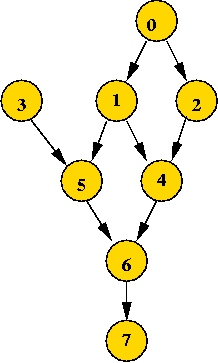• Example application: task scheduling
• A large activity consists of many related tasks.
• Some tasks need to occur before others.
=> typically a precedence relation among tasks.
• Represent task precedence using a DAG.

Sequential scheduling:

• Suppose the tasks represent programs that must be executed on a sequential processor.

• Goal: find an execution sequence that does not violate precedence constraints.

• Example: 3 0 1 2 5 4 6 7

• Note: 4 0 1 2 5 3 6 7 violates precedence requirements.

Topological sort:

• A topological sort of a DAG is a sequence of vertices that:
• does not violate precedence;
• contains all the vertices.

• A simple (the "classic") algorithm:
• Find a vertex that has no predecessors (zero in-degree)
(there must be one, or it's not a DAG).
• Add this to the sequence.
• Remove it from the graph.
• In removing, adjust the in-degree of every neighbor of the removed vertex.
• Repeat.

In-Class Exercise 7.11: Apply the classic algorithm to the above example.

Using depth-first search:

• Key observation: the vertex whose completion occurs first in depth-first search can be placed last in the sequence.

• Why? It has no successors (descendants in the DFS tree).

• Steps:
• Examine completionOrder in depth-first search.
• Place first vertex to complete at end of sequence.
• Remove it from the graph.
• Place next vertex to complete in next-to-last in sequence.
• ... and so on ...

• Note: after DFS "completes" a vertex, the vertex is never seen again
=> no need to remove it from graph!

• Example: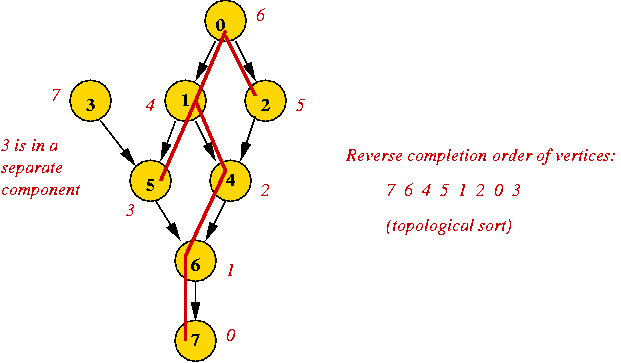Pseudocode:

• We only need to record topological sort order every time a completion occurs.

• Partial pseudocode:
```
Algorithm: depthFirstMatrixRecursive (u, v)
Input: the vertex u from which this is being called, vertex v
to be explored, adjMatrix is assumed to be global.

// Mark vertex v as visited.
1.   visitCount = visitCount + 1
2.   visitOrder[v] = visitCount

// Look for first unvisited neighbor and recurse
3.   for i=0 to n-1
// ... (same as before, not shown) ...
4.   endfor

// After returning from recursion, set completion order:
5 .  completionCount = completionCount + 1
6.   completionOrder[v] = completionCount

// Set topological sort order.
// Initialize topSortCount to -1 outside this method
7.   topSortCount = topSortCount + 1
8.   topSortOrder[topSortCount] = v
```